Function Repository Resource:

# GeneralizedGridGraph

Generates a generalized version of GridGraph

Contributed by: Max Piskunov
 ResourceFunction["GeneralizedGridGraph"][{spec1,spec2,…,speck}] gives a k-dimensional generalized grid graph according to the specifications specj .

## Details and Options

The specj can take any of the following forms:
 m m vertices in the jth dimension m → “Circular” m vertices in the jth dimension connected cyclically m → “Directed” m vertices connected by directed edges in the jth dimension m → {“Circular”, “Directed”} m vertices in the jth dimension cyclically connected by directed edges
GeneralizedGridGraph takes the same options as Graph, with the following additional option:
 “VertexNamingFunction” Automatic function to use for naming the vertices

## Examples

### Basic Examples (2)

Generate an 8×8 grid graph with directed edges:

 In:=Out=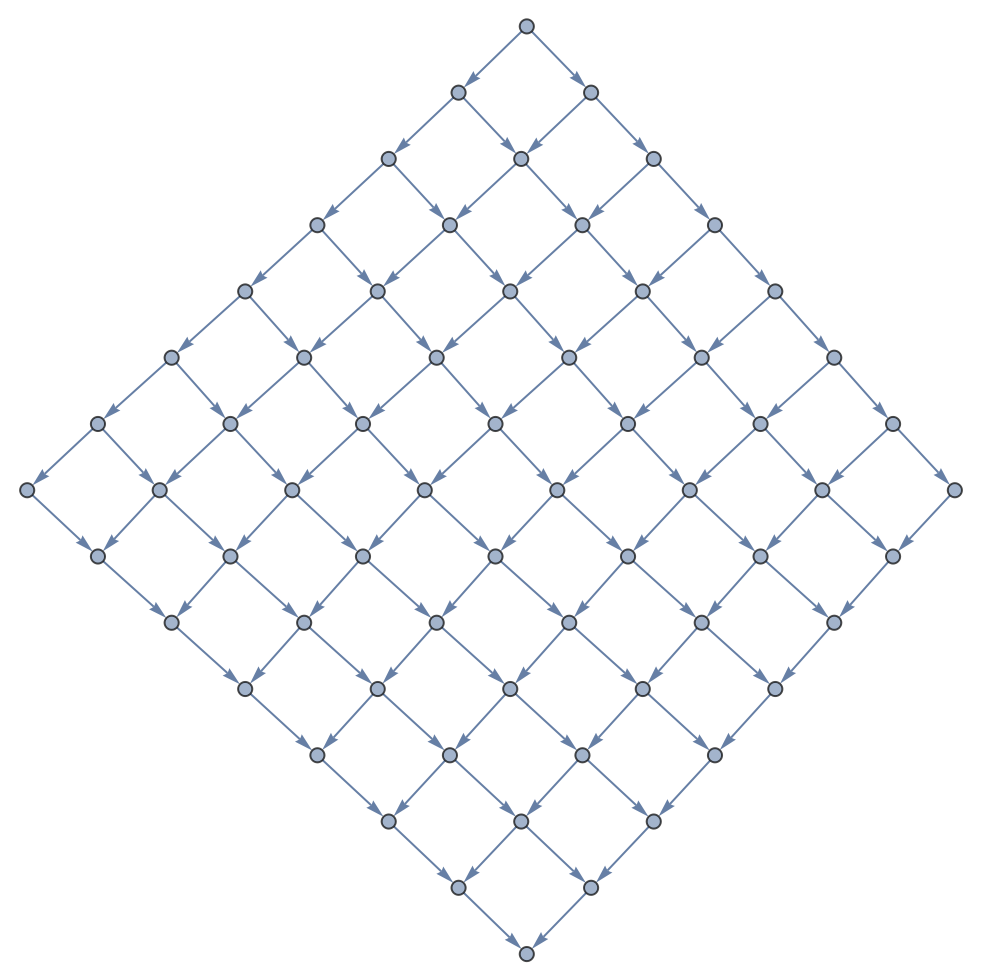Generate an 5×5×5 grid graph with directed edges:

 In:=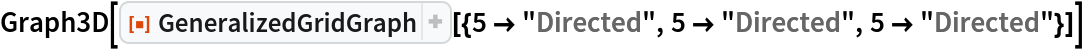Out=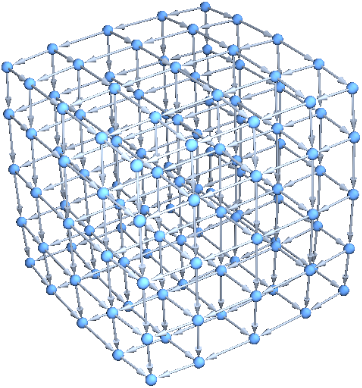### Scope (3)

Generate an ordinary grid graph:

 In:=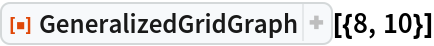Out=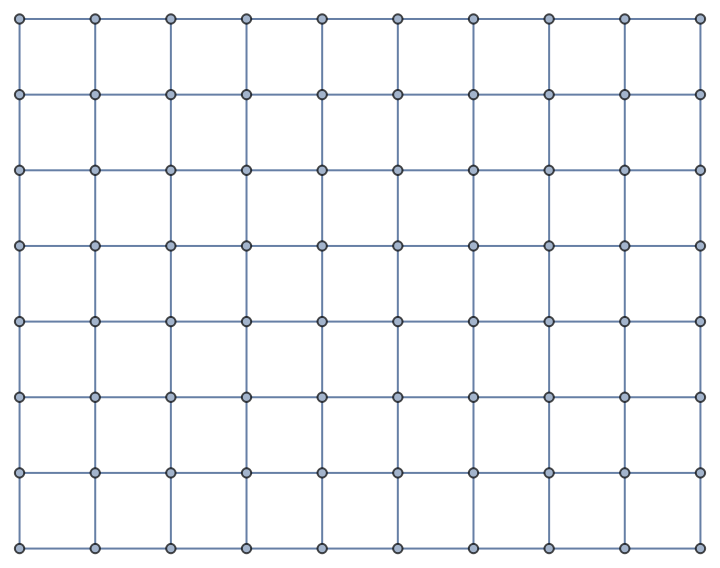Generate a grid graph that is circular in the first dimension, and has directed edges in the second:

 In:=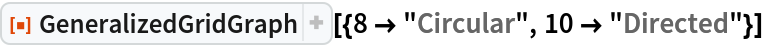Out=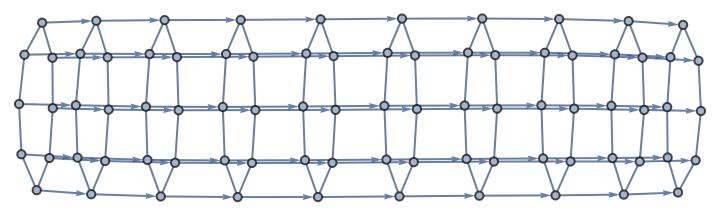Generate a grid graph that has directed edges and is circular in the first dimension:

 In:=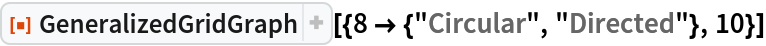Out=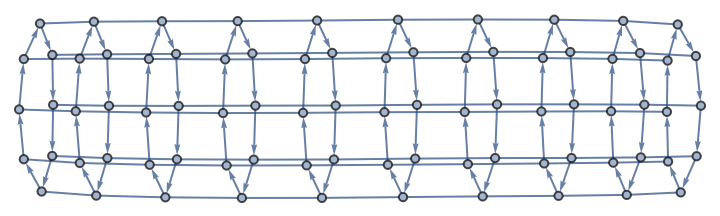### Options (4)

#### EdgeStyle (1)

Give an EdgeStyle:

 In:=Out=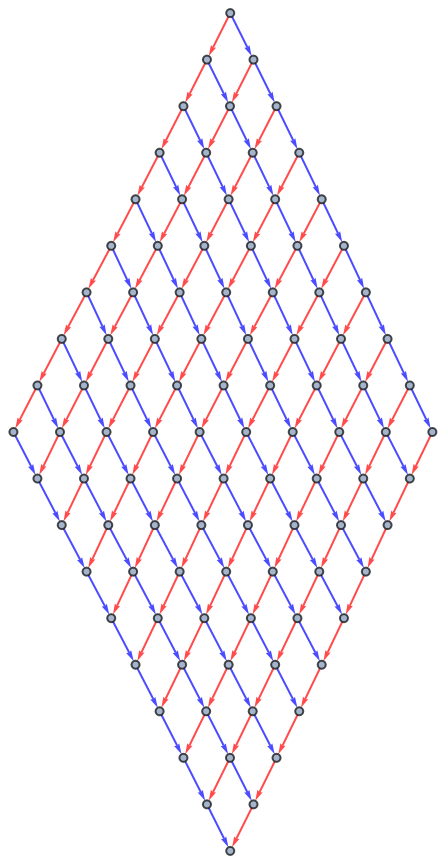In:=Out=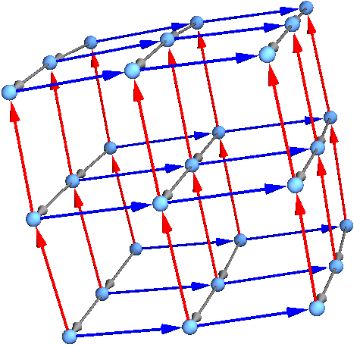#### VertexNamingFunction (3)

Label vertices with their coordinates:

 In:=Out=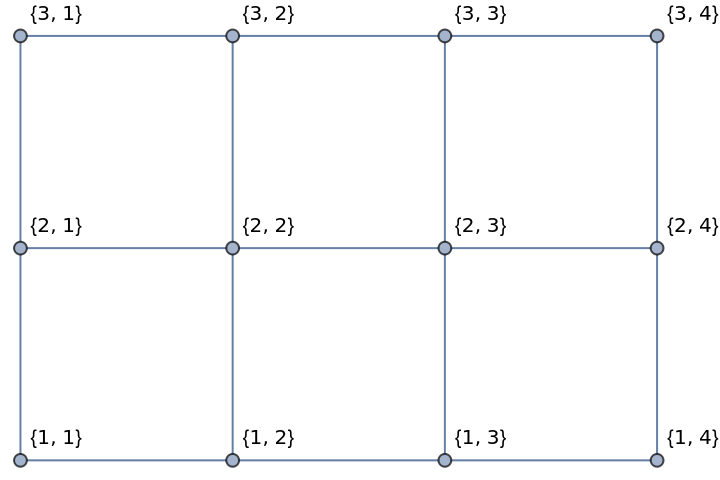Labeling by coordinates works with arbitrary grids:

 In:=Out=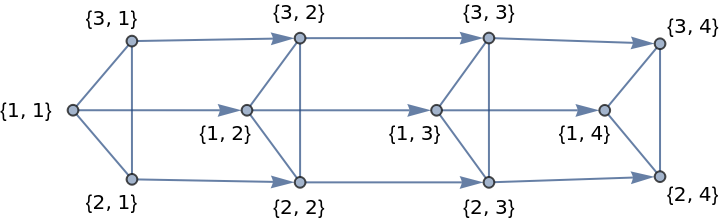Labeling by coordinates works in any dimension:

 In:=Out=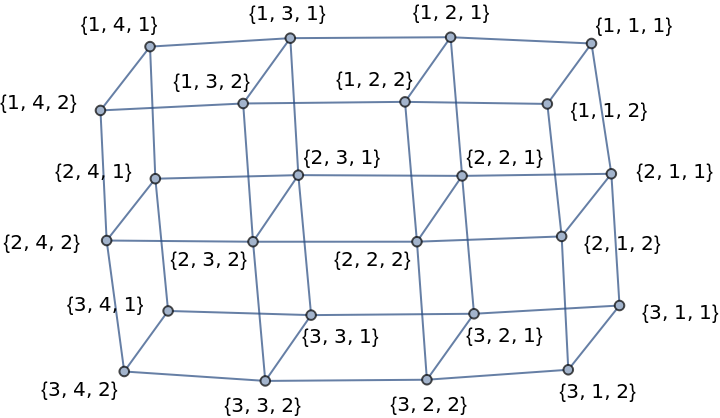### Properties and Relations (1)

For plain integer specifications, GeneralizedGridGraph is the same as GridGraph:

 In:=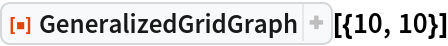Out=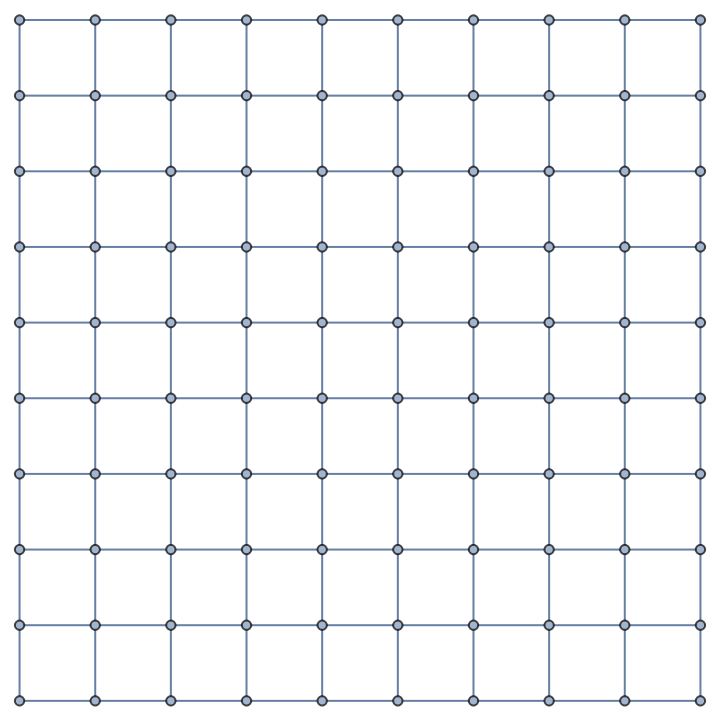In:=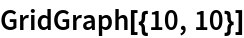Out=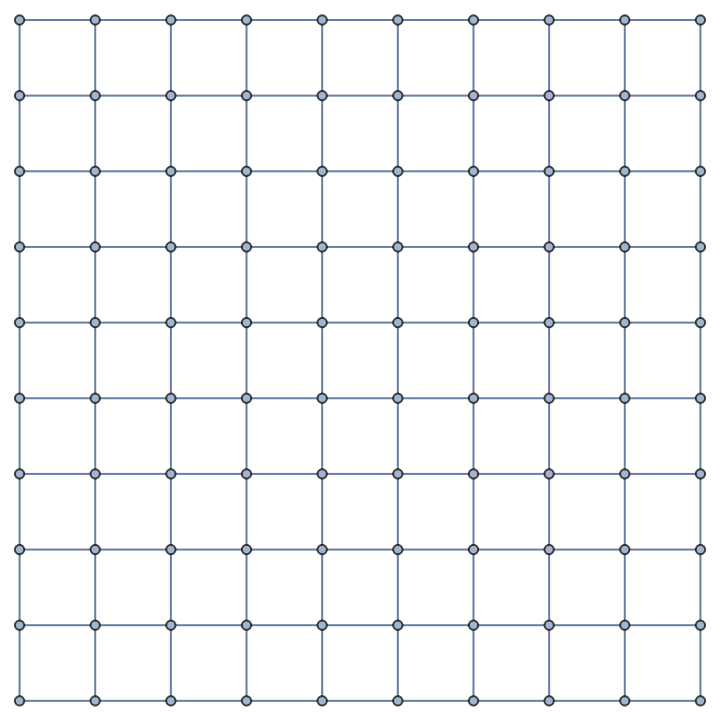## Version History

• 2.0.0 – 16 March 2020
• 1.0.0 – 09 March 2020